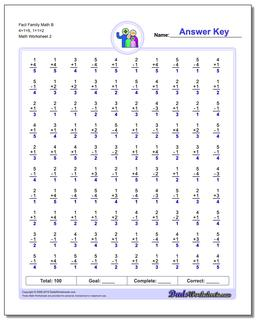# Math Worksheets: Fact Family Math: Fact Family Math: Fact Family Math B 4+1=5, 1+1=2 (Second Worksheet)## Fact Family Math B 4+1=5, 1+1=2 (Second Worksheet)

PropertyValue
DescriptionFact Family Math B 4+1=5, 1+1=2: These math worksheets have 100 addition and subtraction fact family problems and make for a challenging two minute test. (Second Worksheet)
Resource TypeWorksheet